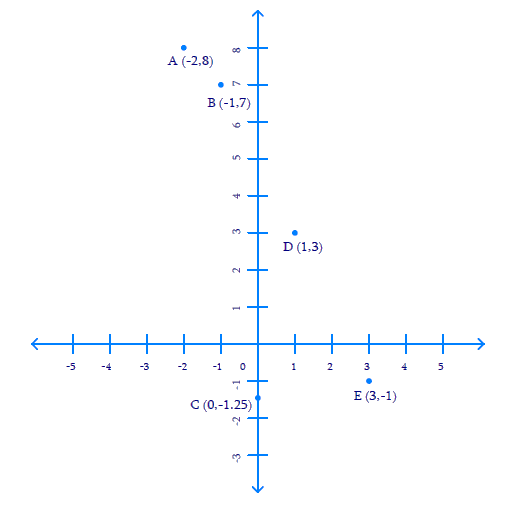# Ex.3.3 Q2 Coordinate Geometry Solution - NCERT Maths Class 9

Go back to  'Ex.3.3'

## Question

Plot the points ($$x, y$$) given in the following table on the plane, choosing suitable units of distance on the axes.

 $${x}$$ $$-2$$ $$-1$$ $$0$$ $$1$$ $$3$$ $$y$$ $$8$$ $$7$$ $$-1.25$$ $$3$$ $$-1$$

Video Solution
Coordinate Geometry
Ex 3.3 | Question 2

## Text Solution

Steps:

Given,

 $$x$$ $$-2$$ $$-1$$ $$0$$ $$1$$ $$3$$ $$y$$ $$8$$ $$7$$ $$-1.25$$ $$3$$ $$-1$$

Draw $$x$$-axis and $$y$$-axis as the co-ordinate axes and mark their point of intersection $$O$$ as the Origin ($$0, 0$$).

In order to plot the points given in the table provided above,

• Let us mark the first point as $$A\,(–2, 8)$$
• Take $$2$$ units on the negative $$x-$$axis and then $$8$$ units on positive $$y-$$axis.
• Let us mark the second point as $$B\,(–1, 7)$$
• Take $$-1$$ unit on negative $$x-$$axis and then $$7$$ units on positive $$y-$$axis.
• Let us mark the third point as $$C\,(0, –1.25),$$
• Take $$1.25$$ units below $$x-$$axis on negative $$y-$$axis.
• Let us mark the fourth point as $$D\,(1, 3)$$
• Take $$1$$ unit on positive $$x-$$axis and then $$3$$ units on positive $$y-$$axis.
• Let us mark the fifth point as $$E\,(3, –1)$$
• Take $$3$$ units on positive $$x-$$axis and then move $$-1$$ unit on negative $$x-$$axis.Video Solution
Coordinate Geometry
Ex 3.3 | Question 2

Learn from the best math teachers and top your exams

• Live one on one classroom and doubt clearing
• Practice worksheets in and after class for conceptual clarity
• Personalized curriculum to keep up with school